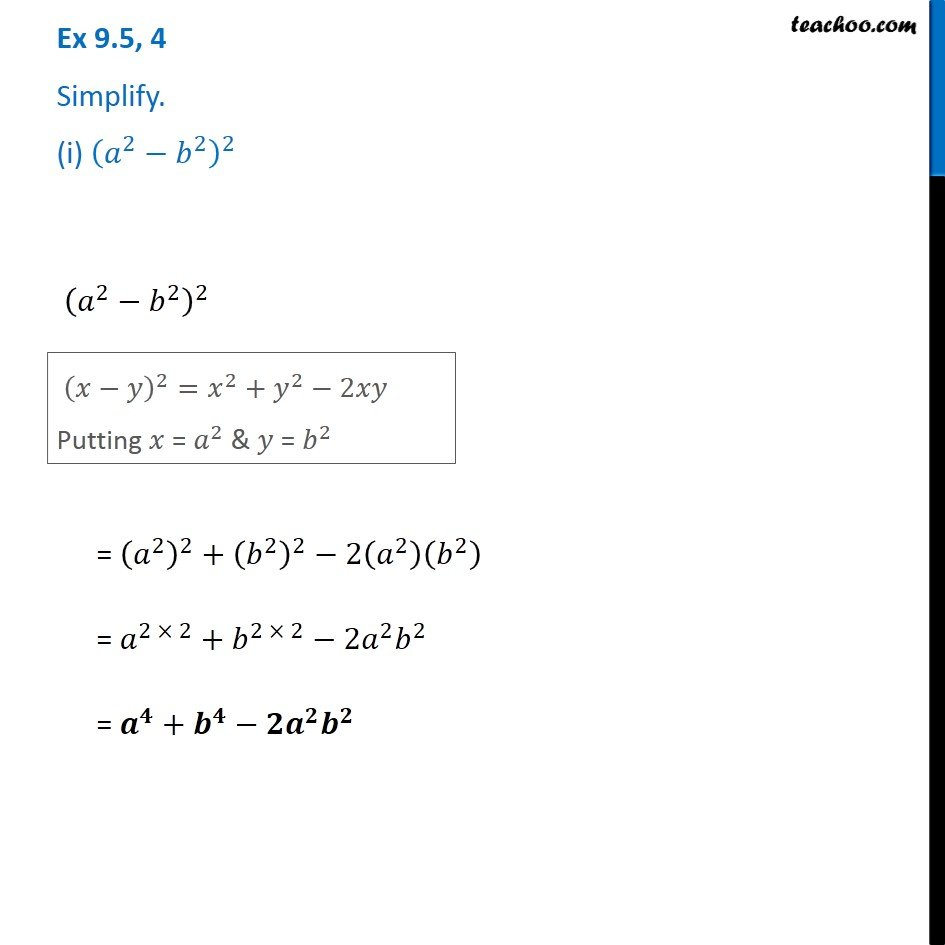Algebra Identities - Identity III

Chapter 8 Class 8 Algebraic Expressions and Identities
Concept wiseLearn in your speed, with individual attention - Teachoo Maths 1-on-1 Class

### Transcript

Question 4 Simplify. (i) (𝑎^2−𝑏^2 )^2 (𝑎^2−𝑏^2 )^2 (𝑥−𝑦)^2=𝑥^2+𝑦^2−2𝑥𝑦 Putting 𝑥 = 𝑎^2 & 𝑦 = 𝑏^2 = (𝑎^2 )^2+(𝑏^2 )^2−2(𝑎^2 )(𝑏^2 ) = 𝑎^(2 × 2)+𝑏^(2 × 2)−2𝑎^2 𝑏^2 = 𝒂^𝟒+𝒃^𝟒−𝟐𝒂^𝟐 𝒃^𝟐